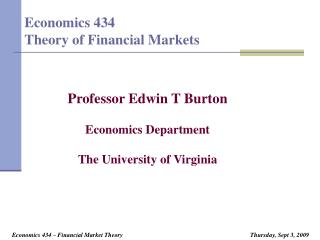DownloadDownload PresentationEconomics 434 Theory of Financial Markets

# Economics 434 Theory of Financial Markets

Télécharger la présentation## Economics 434 Theory of Financial Markets

- - - - - - - - - - - - - - - - - - - - - - - - - - - E N D - - - - - - - - - - - - - - - - - - - - - - - - - - -
##### Presentation Transcript

1. Economics 434Theory of Financial Markets Professor Edwin T Burton Economics Department The University of Virginia

2. Treasury Bills • Anything issued with less than 1 year in original maturity • Everything else is a note or a bond • Three main types • 4 week bill (30 day bill) • 3 month (90 day bill) • 6 month (180 day bill) • Year bill (360 day bill) • Also, from time to time • Cash management bills

3. Key facts about treasury bills • Always have “original maturity” less than one year • Quotes are “discounts” • Year is assumed to be 360 days long • Actual annual yield higher than quote because • Quote is a discount • Year has more days than 360

4. Example: The Year Bill • Assume the quote is 0.40 % • Assume the principal amount is \$ 1 million (always assume this in this class for treasury bills) • What is the price of this bill? • Discount is .004 times \$ 1 million = \$ 4,000 • Price then must be \$ 996,000 • What is the annual yield of this bill? • Yield for 360 days is 4/996 = .004016 % • Yield for 365 days is 365/360 times .004016 % or .004072 or .4072 percent

5. Example: The Three Month Bill • Assume the quote is 0.10 % • Assume the principal amount is \$ 1 million (always assume this in this class for treasury bills) • What is the price of this bill? • Discount is (.001 divided by 4) times \$ 1 million = \$ 250 • Price then must be \$ 999,750 • What is the annual yield of this bill? • Yield for 360 days is 4/996 = .004016 % • Yield for 365 days is 365/360 times .004016 % or .004072 or .4072 percent

6. General Principles • To calculate annual yield • First calculate the amount of the discount • Quote times t/360 times \$ 1 million where is t is the days remaining to maturity • Then note that the amount of the discount is your profit and \$ 1 million minus the discount is your cost, so that the yield for t days is: • Yield for t days = discount/(\$ 1milion – discount) • Then annualize: • Annual yield = (365/t) times (Yield for t days)

7. The End